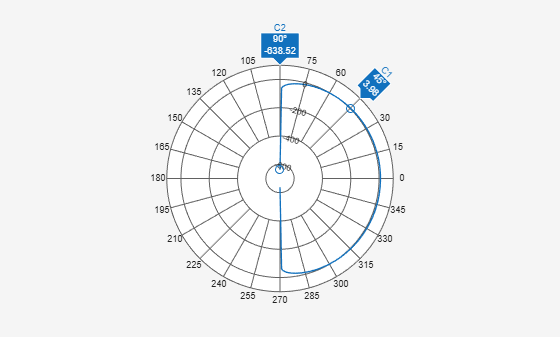Add cursor to polar plot angle

## Syntax

``addCursor(p,angle)``
``addCursor(p,angle,index)``
``id = addCursor(___)``

## Description

example

````addCursor(p,angle)` adds a cursor to the active polar plot, `p`, at the data point closest to the specified `angle`. Angle units are in degrees.The first cursor added is called `'C1'`, the second `'C2'`, and so on.```

example

````addCursor(p,angle,index)` adds a cursor at a specified data set `index`. `index` can be a vector of indices.```
````id = addCursor(___)` returns a cell array with one ID for each cursor created. You can specify any of the arguments from the previous syntaxes.```

## Input Arguments

expand all

Polar plot, specified as a scalar handle.

Angle values at which the cursor is added, specified as a scalar or a vector in degrees.

Data set index, specified as a scalar or a vector.

## Examples

expand all

Create a cosine antenna and plot the polar pattern of its directivity at 75 MHz. Then add cursors at two 150° and 270°.

```cosineantenna = phased.CosineAntennaElement('FrequencyRange',[1.0e0 100.0e9],... 'CosinePower',[2,2]); p = pattern(cosineantenna,75.0e6,[-90:90],0,'Type','Directivity'); P = polarpattern([-90:90],p); addCursor(P,[45 135]);```Create a cosine antenna and plot the polar pattern of its directivity at 75 MHz. Then create an isotropic antenna. Also calculate the directivity of this antenna at 75 MHz. Add the directivity plot of the isotropic antenna to the directivity plot of the cosine antenna. Then add cursors at several points.

```cosineantenna = phased.CosineAntennaElement('FrequencyRange',[1.0e0 100.0e9],... 'CosinePower',[2,2]); p1 = pattern(cosineantenna,75.0e6,[-90:90],0,'Type','Directivity'); P = polarpattern([-90:90],p1); isoantenna = phased.IsotropicAntennaElement('FrequencyRange',... [1.0e0 100.0e9]); p2 = pattern(isoantenna,75.0e6,[-180:180],0,'Type','Directivity'); add(P,[-180:180],p2);```Add a cursor at approximately 30° to the cosine antenna pattern (designated by index 1) and at 150° and 270° to the isotropic polar pattern (designated by index 2).

`addCursor(P,[30.5 149.0 314.7],[1 2 1]);`## Version History

Introduced in R2016a